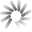## Einsteinium# Einsteinium Hashrate Chart

The Einsteinium hashrate chart provides the current Einsteinium hashrate history in graph format with an option to expand the Einsteinium global hashrate chart time frame back to 2014.

##Loading Einsteinium network hashrate chart

The Einsteinium network hashrate chart can be used to visualize Einsteinium mining hashrate increases and decreases viewable in segment options of daily, weekly, monthly, 3 months, 6 months, 1 year, 3 years, and all time.

## What is Einsteinium Hashrate?

Einsteinium hashrate is a calculated numerical value that specifies an estimate of how many hashes are being generated by Einsteinium miners trying to solve the current Einsteinium block or any given block.

Einsteinium hashrate is represented in Hashes per Second or H/s.

The global Einsteinium network hashrate is a calculated value and is measured in hashes per second (H/s). The calculation uses the current mining difficulty and the average Einsteinium block time between mined blocks versus the defined block time as variables to determine the global Einsteinium network hashrate.

As the Einsteinium network hashrate goes up - the EMC2 hashrate numbers get so large that abbreviations must be used.

The abbreviations are SI derived units representing the number of hashes performed in a one second time frame.

### The current Einsteinium hashrate is 64.76 GH/s, representing the global Einsteinium network hashrate with a mining difficulty of 1.04 K at block height 3,375,563.

Hashrate Unit/s Hash Hashes Per Second
H/s (Hash) 1 One
kH/s (KiloHash) 1,000 One Thousand
MH/s (MegaHash) 1,000,000 One Million
GH/s (GigaHash) 1,000,000,000 One Billion
TH/s (TeraHash) 1,000,000,000,000 One Trillion
EH/s (ExaHash) 1,000,000,000,000,000,000 One Quintillion
ZH/s (ZettaHash) 1,000,000,000,000,000,000,000 One Sextillion
YH/s (YottaHash) 1,000,000,000,000,000,000,000,000 One Septillion

It is important to point out the Einsteinium hashrate does not determine how quickly or slowly each block is solved.

This timing, called the block time is enforced by the Einsteinium mining difficulty value, which is adjusted upwards or downwards during each block difficulty retarget to keep blocks being solved at a constant time frame.

You can calculate Einsteinium mining profits using the current EMC2 hashrate difficulty and our Einsteinium mining calculator.

## What is the Current Einsteinium Hashrate?

The current Einsteinium hashrate (EMC2 hashrate) is 64.76 GH/s at block height 3,375,563 with a difficulty of 1,036.07.

Einsteinium Hashrate Stats

### Current Einsteinium Hashrate

Einsteinium Global Hashrate
64.76 GH/s

### Einsteinium Hashrate All Time High

Einsteinium Hashrate on Oct 09, 2017 at block 1,616,617
2.27 TH/s

## How to Calculate Einsteinium Hashrate

The Einsteinium hashrate is calculated using the current Einsteinium difficulty, the defined Einsteinium block time, and the average block time of the last (X) number of blocks.

Most full Einsteinium nodes will have an option to see the current Einsteinium global hashrate using the "getnetworkhashps" command in the console window.Given the fact that the Einsteinium difficulty value determines statically how many hashes are needed to solve the next block, it is recommended to use the current mining difficulty to determine the statistically accurate number of Einsteinium that can be mined in a given time frame.

Once again we recommend using our Einsteinium mining calculator as the current Einsteinium difficulty is preloaded, along with the latest Einsteinium price.

\$0.0435
\$0.00 (3.26 %)

24 hour change